##### Chemistry Workbook For Dummies with Online Practice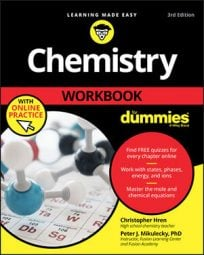In real-life chemical reactions, not all of the reactants (substances present at the start of a chemical reaction) convert into product. More typically, one reagent (what is added to cause or test for a chemical reaction) is completely used up, and others are left in excess, perhaps to react another day. The reactant that is used up is the limiting reagent.

Chemists need to know which reactant will run out first, because that information allows them to deduce how much product and excess reagent they can expect, based on how much of the limiting reagent they've put into the reaction.

In any chemical reaction, you can simply pick one reagent as a candidate for the limiting reagent, calculate how many moles of that reagent you have, and then calculate how many grams of the other reagent you'd need to react both to completion. You'll discover one of two things: either you have an excess of the first reagent, or you have an excess of the second reagent. The one you have in excess is the excess reagent. The one that isn't in excess is the limiting reagent.

Here's an example. Say you are conducting an experiment where ammonia reacts with oxygen to produce nitrogen monoxide and liquid water: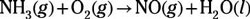In order to find the limiting reagents, excess reagents, and products in this reaction, you need to do the following:

1. Balance the equation.

2. Determine the limiting reagent if 100 g of each reagent are present at the beginning of the reaction.

3. Identify the excess reagent, as well as how many grams of the excess reagent will remain when the reaction reaches completion.

4. Calculate how many grams of each product will be produced if the reaction goes to completion.

So, here's the solution:
1. Balance the equation.

Before doing anything else, you must have a balanced reaction equation. Don't waste time or good thought on an unbalanced equation. The balanced form of the given equation is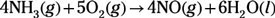Two candidates, NH3 and O2, vie for the status of limiting reagent. You start with 100 g of each, which corresponds to some number of moles of each. Furthermore, you can tell from the coefficients in the balanced equation this reaction requires 4 mol of ammonia for every 5 mol of oxygen gas.

2. Determine the limiting reagent if 100 g of ammonia and 100 g of oxygen are present at the beginning of the reaction.

To find the limiting reactant, you simply need to perform a mass-to-mass (gram-to-gram) calculation from one reactant to the other. This allows you to see which reactant runs out first. You can start with either reactant and convert to mass of the other. In this example, let's start with ammonia: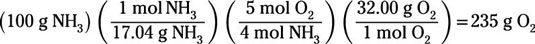The calculation reveals that you'd need 235 g of oxygen gas to completely react with 100 g of ammonia. But you have only 100 g of oxygen. You'll run out of oxygen before you run out of ammonia, so oxygen is the limiting reagent.

3. Identify the excess reagent, as well as how many grams of the excess reagent will remain when the reaction reaches completion.

To calculate how many grams of ammonia will be left at the end of the reaction, assume that all 100 g of oxygen react: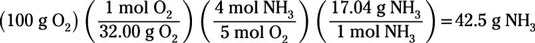This calculation shows that 42.5 g of the original 100 g of ammonia will react before the limiting reagent is expended. So, the excess reagent is ammonia, and 57.5 g of ammonia will remain when the reaction reaches completion (just subtract 42.5 from 100).

4. Calculate how many grams of nitrogen monoxide and water will be produced if the reaction goes to completion.

This problem asks how much of a product is produced. For this calculation, you must begin with the limiting reactant. To determine the grams of nitrogen monoxide that are generated by the complete reaction of oxygen, start with the assumption that all 100 g of the oxygen react: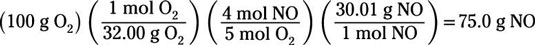So, 75 g of nitrogen monoxide will be produced.

Again, assume that all 100 g of the oxygen react in order to determine how many grams of water are produced: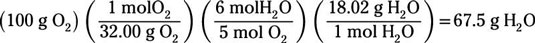You find that 67.5g of water will be produced.